# How to do a t-test or ANOVA for more than one variable at once in R# Introduction

As part of my teaching assistant position in a Belgian university, students often ask me for some help in their statistical analyses for their master’s thesis.

A frequent question is how to compare groups of patients in terms of several quantitative continuous variables. Most of us know that:

• To compare two groups, a Student’s t-test should be used1
• To compare three groups or more, an ANOVA should be performed

These two tests are quite basic and have been extensively documented online and in statistical textbooks so the difficulty is not in how to perform these tests.

In the past, I used to do the analyses by following these 3 steps:

1. Draw boxplots illustrating the distributions by group (with the boxplot() function or thanks to the {esquisse} R Studio addin if I wanted to use the {ggplot2} package)
2. Perform a t-test or an ANOVA depending on the number of groups to compare (with the t.test() and oneway.test() functions for t-test and ANOVA, respectively)
3. Repeat steps 1 and 2 for each variable

This was feasible as long as there were only a couple of variables to test. Nonetheless, most students came to me asking to perform these kind of tests not on one or two variables, but on multiples variables. So when there were more than one variable to test, I quickly realized that I was wasting my time and that there must be a more efficient way to do the job.

Note: you must be very careful with the issue of multiple testing (also referred as multiplicity) which can arise when you perform multiple t-tests. In short, when a large number of statistical tests are performed, some will have p-values less than 0.05 purely by chance, even if all null hypotheses are in fact really true. This is known as multiplicity or multiple testing. You can tackle this problem by using the Bonferroni correction, among others. The Bonferroni correction is a simple method that allows many t-tests to be made while still assuring an overall confidence level is maintained. For this, instead of using the standard threshold of $$\alpha = 5$$% for the significance level, you can use $$\alpha = \frac{0.05}{m}$$ where $$m$$ is the number of t-tests. For example, if you perform 20 t-tests with a desired $$\alpha = 0.05$$, the Bonferroni correction implies that you would reject the null hypothesis for each individual test when the p-value is smaller than $$\alpha = \frac{0.05}{20} = 0.0025$$.

Note also that there is no universally accepted approach for dealing with the problem of multiple comparisons. Usually, you should choose a p-value adjustment measure familiar to your audience or in your field of study. The Bonferroni correction is the most common way to take this into account and is easy to implement. It is however not appropriate if you have a very large number of tests to perform (imagine you want to do 10,000 t-tests, a p-value would have to be less than $$\frac{0.05}{10000} = 0.000005$$ to be significant). A more powerful method is also to adjust the false discovery rate using the Benjamini-Hochberg procedure (McDonald, 2014). This article aims at presenting a way to perform multiple t-tests and ANOVA from a technical point of view (how to implement it in R). Discussion on which adjustment method to use or whether there is a more appropriate model to fit the data is beyond the scope of this article (so be sure to understand the implications of using the code below for your own analyses). Make sure also to test the assumptions of the ANOVA before interpreting results.

# Perform multiple tests at once

I thus wrote a piece of code that automated the process, by drawing boxplots and performing the tests on several variables at once. Below is the code I used, illustrating the process with the iris dataset. The Species variable has 3 levels, so let’s remove one, and then draw a boxplot and apply a t-test on all 4 continuous variables at once. Note that the continuous variables that we would like to test are variables 1 to 4 in the iris dataset.

dat <- iris

# remove one level to have only two groups
dat <- subset(dat, Species != "setosa")
dat$Species <- factor(dat$Species)

# boxplots and t-tests for the 4 variables at once
for (i in 1:4) { # variables to compare are variables 1 to 4
boxplot(dat[, i] ~ dat$Species, # draw boxplots by group ylab = names(dat[i]), # rename y-axis with variable's name xlab = "Species" ) print(t.test(dat[, i] ~ dat$Species)) # print results of t-test
}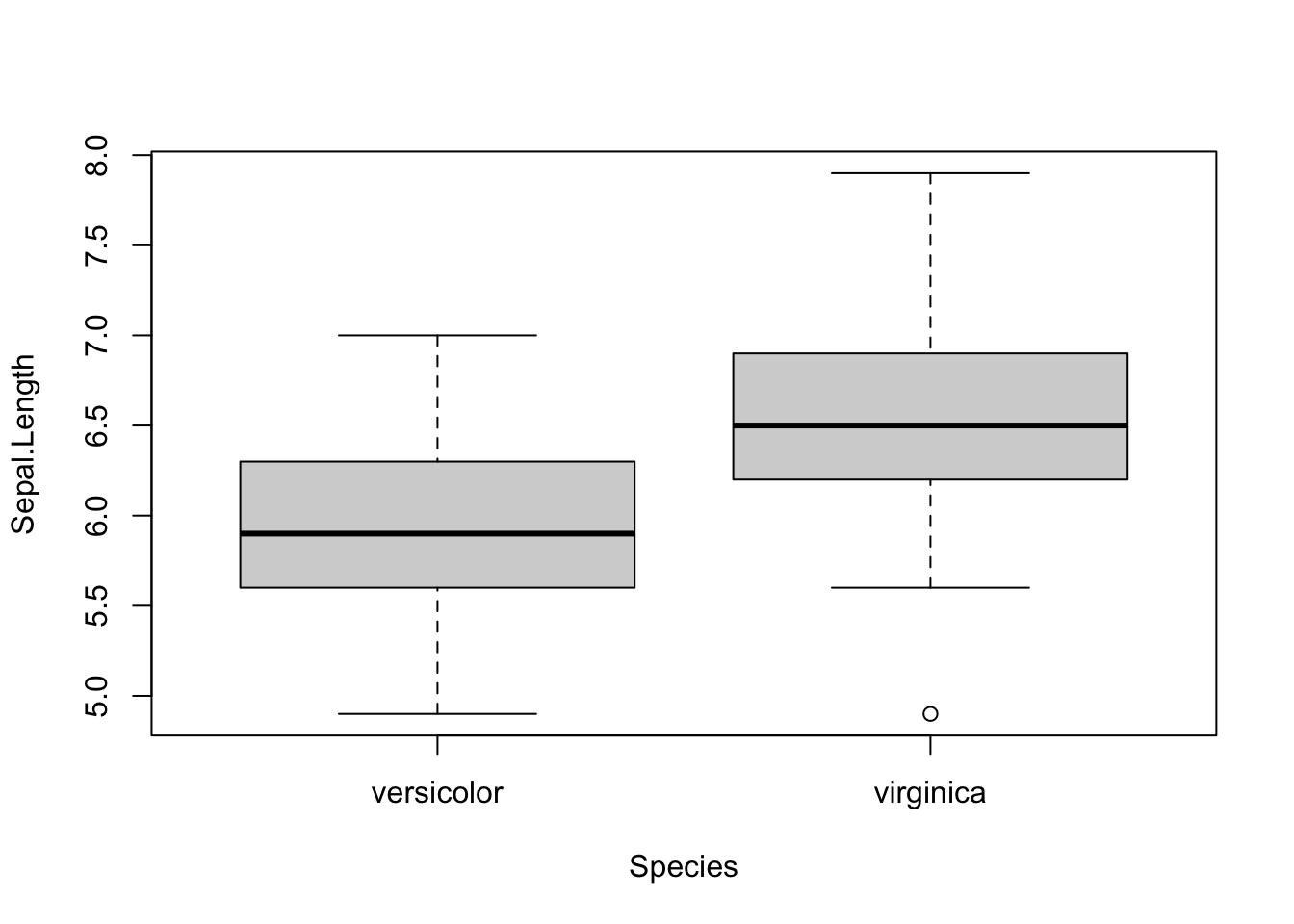##
##  Welch Two Sample t-test
##
## data:  dat[, i] by dat$Species ## t = -5.6292, df = 94.025, p-value = 1.866e-07 ## alternative hypothesis: true difference in means is not equal to 0 ## 95 percent confidence interval: ## -0.8819731 -0.4220269 ## sample estimates: ## mean in group versicolor mean in group virginica ## 5.936 6.588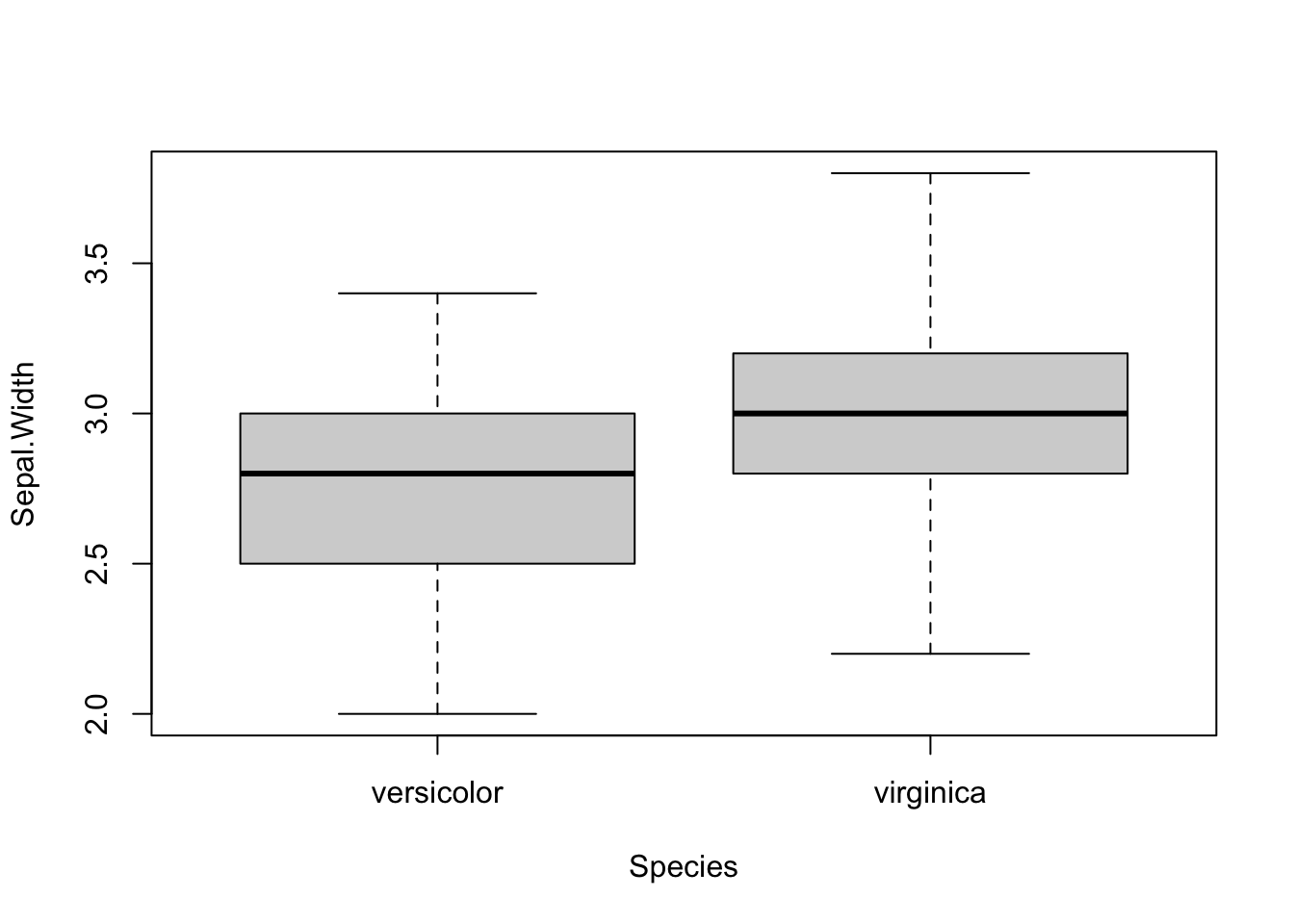## ## Welch Two Sample t-test ## ## data: dat[, i] by dat$Species
## t = -3.2058, df = 97.927, p-value = 0.001819
## alternative hypothesis: true difference in means is not equal to 0
## 95 percent confidence interval:
##  -0.33028364 -0.07771636
## sample estimates:
## mean in group versicolor  mean in group virginica
##                    2.770                    2.974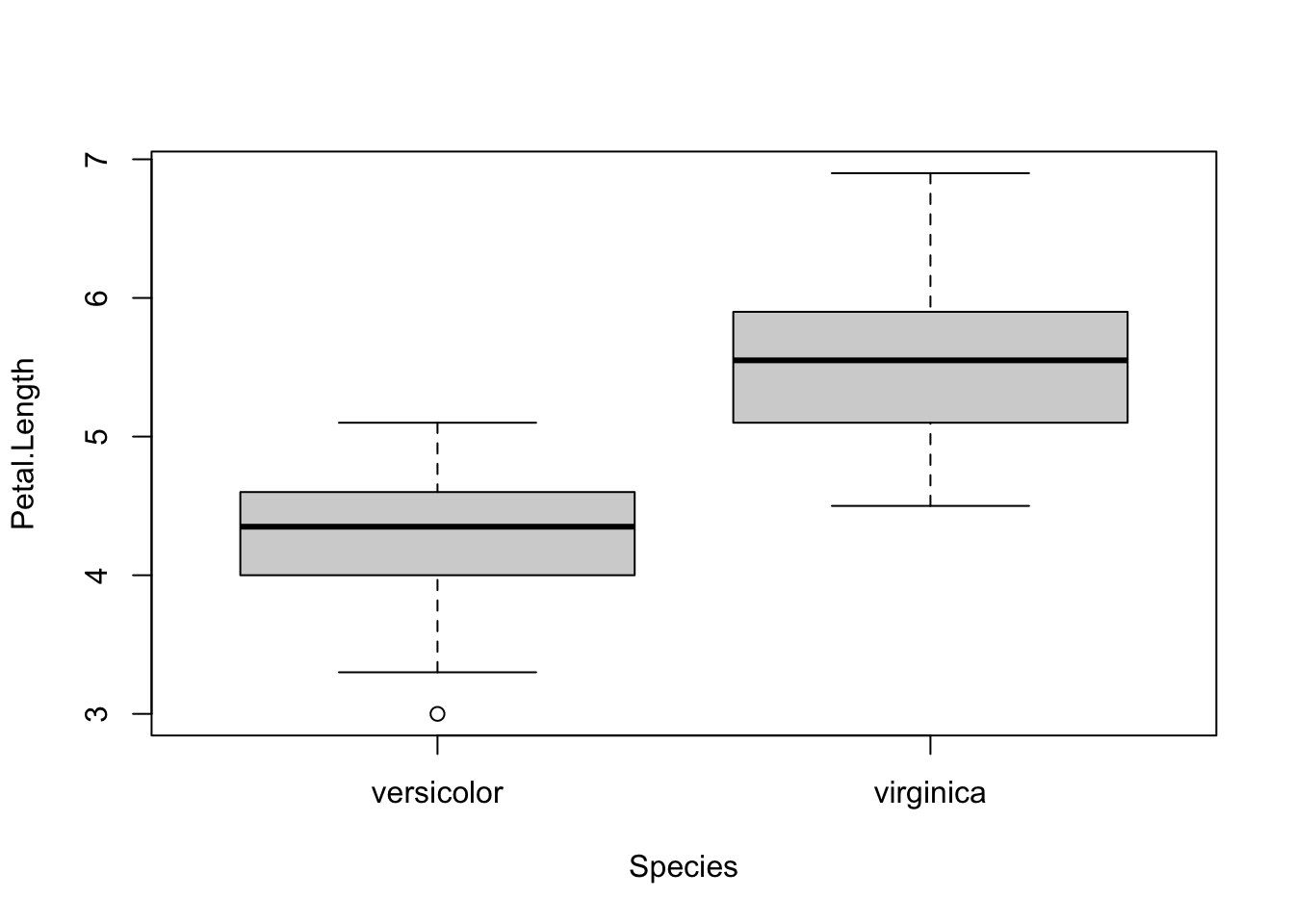##
##  Welch Two Sample t-test
##
## data:  dat[, i] by dat$Species ## t = -12.604, df = 95.57, p-value < 2.2e-16 ## alternative hypothesis: true difference in means is not equal to 0 ## 95 percent confidence interval: ## -1.49549 -1.08851 ## sample estimates: ## mean in group versicolor mean in group virginica ## 4.260 5.552## ## Welch Two Sample t-test ## ## data: dat[, i] by dat$Species
## t = -14.625, df = 89.043, p-value < 2.2e-16
## alternative hypothesis: true difference in means is not equal to 0
## 95 percent confidence interval:
##  -0.7951002 -0.6048998
## sample estimates:
## mean in group versicolor  mean in group virginica
##                    1.326                    2.026

As you can see, the above piece of code draws a boxplot and then prints results of the test for each continuous variable, all at once.

At some point in the past, I even wrote code to:

1. draw a boxplot
2. test for the equality of variances (thanks to the Levene’s test)
3. depending on whether the variances were equal or unequal, the appropriate test was applied: the Welch test if the variances were unequal and the Student’s t-test in the case the variances were equal (see more details about the different versions of the t-test for two samples)
4. apply steps 1 to 3 for all continuous variables at once

I had a similar code for ANOVA in case I needed to compare more than two groups.

The code was doing the job relatively well. Indeed, thanks to this code I was able to test several variables in an automated way in the sense that it compared groups for all variables at once.

The only thing I had to change from one project to another is that I needed to modify the name of the grouping variable and the numbering of the continuous variables to test (Species and 1:4 in the above code).

# Concise and easily interpretable results

## T-test

Although it was working quite well and applicable to different projects with only minor changes, I was still unsatisfied with another point.

Someone who is proficient in statistics and R can read and interpret the output of a t-test without any difficulty. However, as you may have noticed with your own statistical projects, most people do not know what to look for in the results and are sometimes a bit confused when they see so many graphs, code, output, results and numeric values in a document. They are quite easily overwhelmed by this mass of information.

With my old R routine, the time I was saving by automating the process of t-tests and ANOVA was (partially) lost when I had to explain R outputs to my students so that they could interpret the results correctly. Although most of the time it simply boiled down to pointing out what to look for in the outputs (i.e., p-values), I was still losing quite a lot of time because these outputs were, in my opinion, too detailed for most real-life applications. In other words, too much information seemed to be confusing for many people so I was still not convinced that it was the most optimal way to share statistical results to nonscientists.

Of course, they came to me for statistical advices, so they expected to have these results and I needed to give them answers to their questions and hypotheses. Nonetheless, I wanted to find a better way to communicate these results to this type of audience, with the minimum of information required to arrive at a conclusion. No more and no less than that.

After a long time spent online trying to figure out a way to present results in a more concise and readable way, I discovered the {ggpubr} package. This package allows to indicate the test used and the p-value of the test directly on a ggplot2-based graph. It also facilitates the creation of publication-ready plots for non-advanced statistical audiences.

After many refinements and modifications of the initial code (available in this article), I finally came up with a rather stable and robust process to perform t-tests and ANOVA for more than one variable at once, and more importantly, make the results concise and easily readable by anyone (statisticians or not).

A graph is worth a thousand words, so here are the exact same tests than in the previous section, but this time with my new R routine:

library(ggpubr)

# Edit from here #
x <- which(names(dat) == "Species") # name of grouping variable
y <- which(names(dat) == "Sepal.Length" # names of variables to test
| names(dat) == "Sepal.Width"
| names(dat) == "Petal.Length"
| names(dat) == "Petal.Width")
method <- "t.test" # one of "wilcox.test" or "t.test"
paired <- FALSE # if paired make sure that in the dataframe you have first all individuals at T1, then all individuals again at T2
# Edit until here

# Edit at your own risk
for (i in y) {
for (j in x) {
ifelse(paired == TRUE,
p <- ggpaired(dat,
x = colnames(dat[j]), y = colnames(dat[i]),
color = colnames(dat[j]), line.color = "gray", line.size = 0.4,
palette = "npg",
legend = "none",
xlab = colnames(dat[j]),
ylab = colnames(dat[i]),
),
p <- ggboxplot(dat,
x = colnames(dat[j]), y = colnames(dat[i]),
color = colnames(dat[j]),
palette = "npg",
legend = "none",
)
)
print(p + stat_compare_means(aes(label = paste0(..method.., ", p-value = ", ..p.format..)),
method = method,
paired = paired,
# group.by = NULL,
ref.group = NULL
))
}
}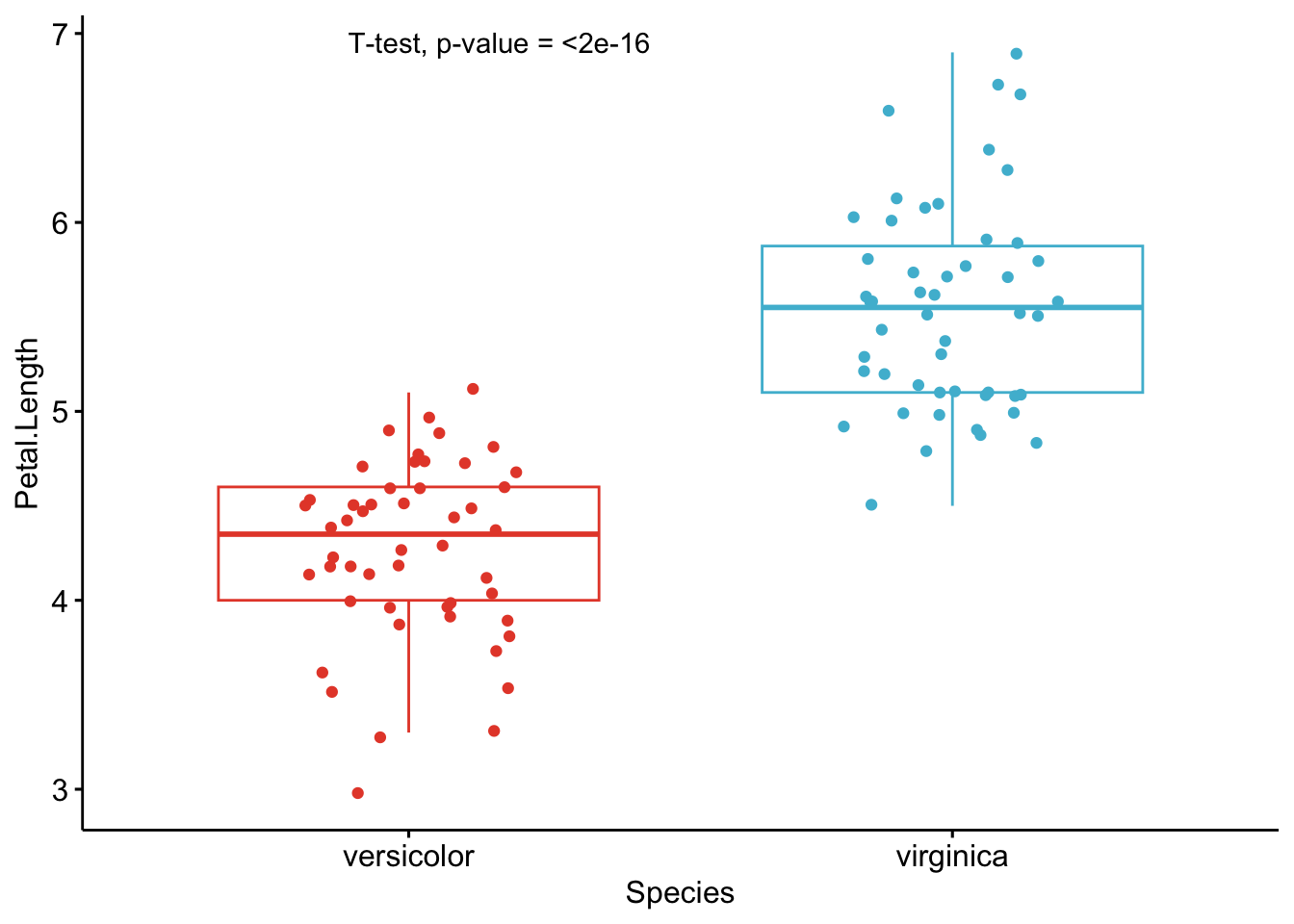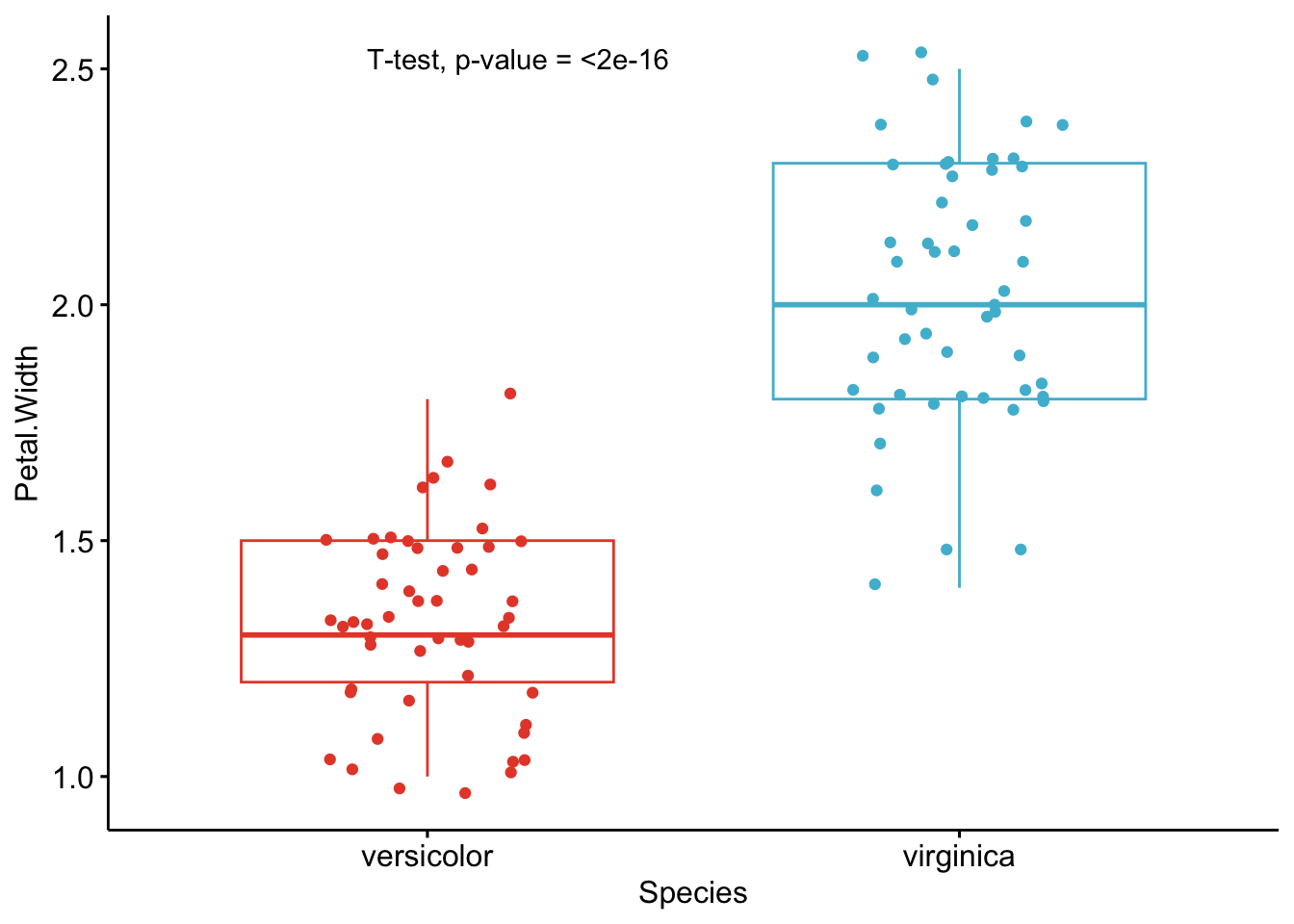As you can see from the graphs above, only the most important information is presented for each variable:

• a visual comparison of the groups thanks to boxplots
• the name of the statistical test
• the p-value of the test

Of course, experts may be interested in more advanced results. However, this simple yet complete graph, which includes the name of the test and the p-value, gives all the necessary information to answer the question: “Are the groups different?”.

In my experience, I have noticed that students and professionals (especially those from a less scientific background) understand way better these results than the ones presented in the previous section.

The only lines of code that need to be modified for your own project is the name of the grouping variable (Species in the above code), the names of the variables you want to test (Sepal.Length, Sepal.Width, etc.),2 whether you want to apply a t-test (t.test) or Wilcoxon test (wilcox.test) and whether the samples are paired or not (FALSE if samples are independent, TRUE if they are paired).

Based on these graphs, it is easy, even for non-experts, to interpret the results and conclude that the versicolor and virginica species are significantly different in terms of all 4 variables (since all p-values $$< \frac{0.05}{4} = 0.0125$$ (remind that the Bonferroni correction is applied to avoid the issue of multiple testing, so we divide the usual $$\alpha$$ level by 4 because there are 4 t-tests)).

If you would like to use another p-value adjustment method, you can use the p.adjust() function. Below are the raw p-values found above, together with p-values derived from the main adjustment methods (presented in a dataframe):

raw_pvalue <- numeric(length = length(1:4))
for (i in (1:4)) {
raw_pvalue[i] <- t.test(dat[, i] ~ dat$Species, paired = FALSE, alternative = "two.sided" )$p.value
}

df <- data.frame(
Variable = names(dat[, 1:4]),
raw_pvalue = round(raw_pvalue, 3)
)

df$Bonferroni <- p.adjust(df$raw_pvalue,
method = "bonferroni"
)
df$BH <- p.adjust(df$raw_pvalue,
method = "BH"
)
df$Holm <- p.adjust(df$raw_pvalue,
method = "holm"
)
df$Hochberg <- p.adjust(df$raw_pvalue,
method = "hochberg"
)
df$Hommel <- p.adjust(df$raw_pvalue,
method = "hommel"
)
df$BY <- round(p.adjust(df$raw_pvalue,
method = "BY"
), 3)
df
##       Variable raw_pvalue Bonferroni    BH  Holm Hochberg Hommel    BY
## 1 Sepal.Length      0.000      0.000 0.000 0.000    0.000  0.000 0.000
## 2  Sepal.Width      0.002      0.008 0.002 0.002    0.002  0.002 0.004
## 3 Petal.Length      0.000      0.000 0.000 0.000    0.000  0.000 0.000
## 4  Petal.Width      0.000      0.000 0.000 0.000    0.000  0.000 0.000

Regardless of the p-value adjustment method, the two species are different for all 4 variables. Note that the adjustment method should be chosen before looking at the results to avoid choosing the method based on the results.

Below another function that allows to perform multiple Student’s t-tests or Wilcoxon tests at once and choose the p-value adjustment method. The function also allows to specify whether samples are paired or unpaired and whether the variances are assumed to be equal or not. (The code has been adapted from Mark White’s article.)

t_table <- function(data, dvs, iv,
var_equal = TRUE,
alpha = 0.05,
paired = FALSE,
wilcoxon = FALSE) {
if (!inherits(data, "data.frame")) {
stop("data must be a data.frame")
}

if (!all(c(dvs, iv) %in% names(data))) {
stop("at least one column given in dvs and iv are not in the data")
}

if (!all(sapply(data[, dvs], is.numeric))) {
stop("all dvs must be numeric")
}

if (length(unique(na.omit(data[[iv]]))) != 2) {
stop("independent variable must only have two unique values")
}

out <- lapply(dvs, function(x) {
if (paired == FALSE & wilcoxon == FALSE) {
tres <- t.test(data[[x]] ~ data[[iv]], var.equal = var_equal)
}

else if (paired == FALSE & wilcoxon == TRUE) {
tres <- wilcox.test(data[[x]] ~ data[[iv]])
}

else if (paired == TRUE & wilcoxon == FALSE) {
tres <- t.test(data[[x]] ~ data[[iv]],
var.equal = var_equal,
paired = TRUE
)
}

else {
tres <- wilcox.test(data[[x]] ~ data[[iv]],
paired = TRUE
)
}

c(
p_value = tres$p.value ) }) out <- as.data.frame(do.call(rbind, out)) out <- cbind(variable = dvs, out) names(out) <- gsub("[^0-9A-Za-z_]", "", names(out)) out$p_value <- ifelse(out$p_value < 0.001, "<0.001", round(p.adjust(out$p_value, p_adj), 3)
)
out$conclusion <- ifelse(out$p_value < alpha,
paste0("Reject H0 at ", alpha * 100, "%"),
paste0("Do not reject H0 at ", alpha * 100, "%")
)

return(out)
}

Applied to our dataset, with no adjustment method for the p-values:

result <- t_table(
data = dat,
c("Sepal.Length", "Sepal.Width", "Petal.Length", "Petal.Width"),
"Species"
)

result
##       variable p_value      conclusion
## 1 Sepal.Length  <0.001 Reject H0 at 5%
## 2  Sepal.Width   0.002 Reject H0 at 5%
## 3 Petal.Length  <0.001 Reject H0 at 5%
## 4  Petal.Width  <0.001 Reject H0 at 5%

And with the Holm (1979) adjustment method:

result <- t_table(
data = dat,
c("Sepal.Length", "Sepal.Width", "Petal.Length", "Petal.Width"),
"Species",
)

result
##       variable p_value      conclusion
## 1 Sepal.Length  <0.001 Reject H0 at 5%
## 2  Sepal.Width   0.002 Reject H0 at 5%
## 3 Petal.Length  <0.001 Reject H0 at 5%
## 4  Petal.Width  <0.001 Reject H0 at 5%

Again, with the Holm’s adjustment method, we conclude that, at the 5% significance level, the two species are significantly different from each other in terms of all 4 variables.

## ANOVA

Below the same process with an ANOVA. Note that we reload the dataset iris to include all three Species this time:

dat <- iris

# Edit from here
x <- which(names(dat) == "Species") # name of grouping variable
y <- which(names(dat) == "Sepal.Length" # names of variables to test
| names(dat) == "Sepal.Width"
| names(dat) == "Petal.Length"
| names(dat) == "Petal.Width")
method1 <- "anova" # one of "anova" or "kruskal.test"
method2 <- "t.test" # one of "wilcox.test" or "t.test"
my_comparisons <- list(c("setosa", "versicolor"), c("setosa", "virginica"), c("versicolor", "virginica")) # comparisons for post-hoc tests
# Edit until here

# Edit at your own risk
for (i in y) {
for (j in x) {
p <- ggboxplot(dat,
x = colnames(dat[j]), y = colnames(dat[i]),
color = colnames(dat[j]),
legend = "none",
palette = "npg",
)
print(
p + stat_compare_means(aes(label = paste0(..method.., ", p-value = ", ..p.format..)),
method = method1, label.y = max(dat[, i], na.rm = TRUE)
)
+ stat_compare_means(comparisons = my_comparisons, method = method2, label = "p.format") # remove if p-value of ANOVA or Kruskal-Wallis test >= alpha
)
}
}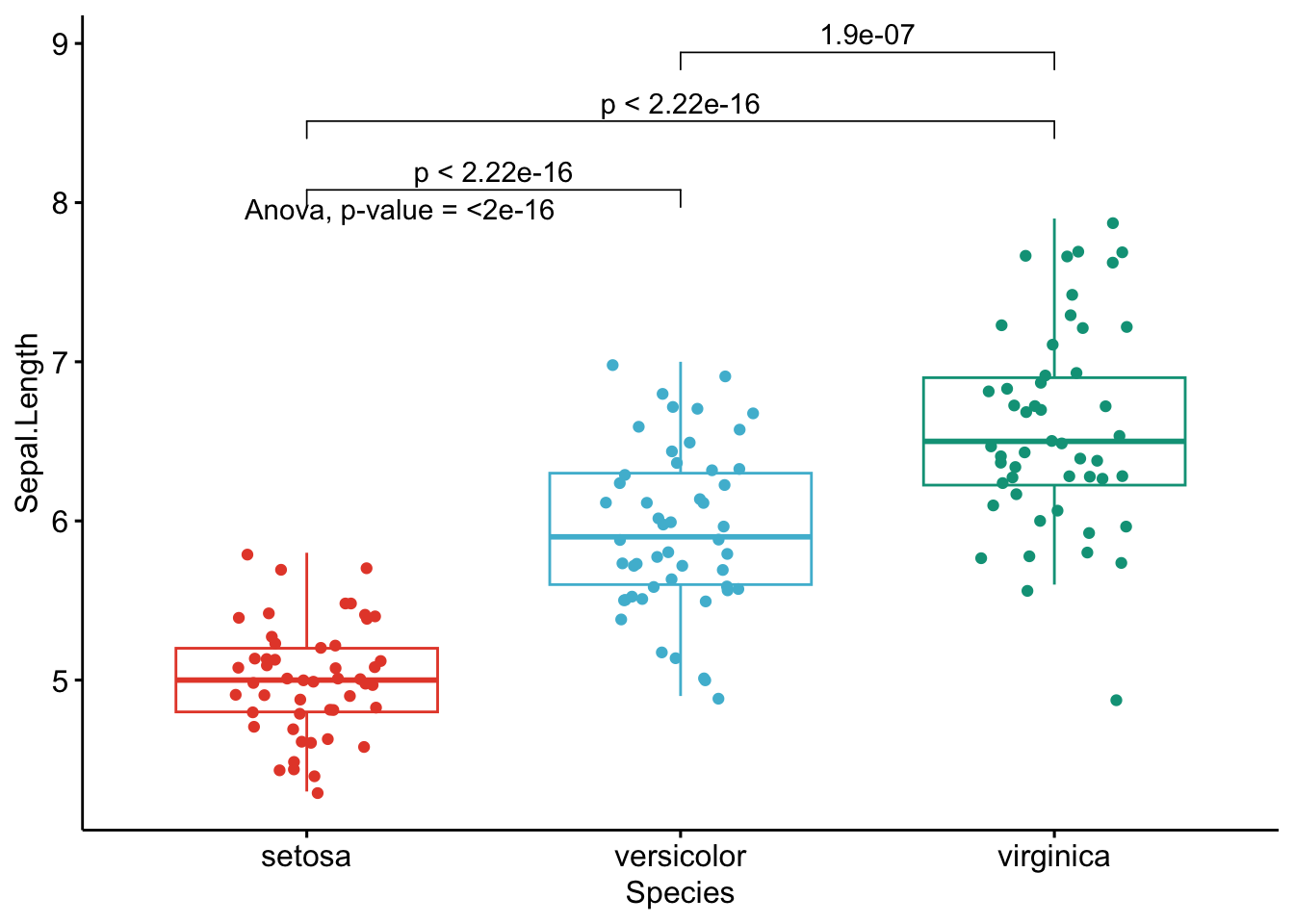Like the improved routine for the t-test, I have noticed that students and non-expert professionals understand ANOVA results presented this way much more easily compared to the default R outputs.

With one graph for each variable, it is easy to see that all species are different from each other in terms of all 4 variables.

If you want to apply the same automated process to your data, you will need to modify the name of the grouping variable (Species), the names of the variables you want to test (Sepal.Length, etc.), whether you want to perform an ANOVA (anova) or Kruskal-Wallis test (kruskal.test) and finally specify the comparisons for the post-hoc tests.3

# To go even further

As we have seen, these two improved R routines allow to:

1. Perform t-tests and ANOVA on a small or large number of variables with only minor changes to the code. I basically only have to replace the variable names and the name of the test I want to use. It takes almost the same time to test one or several variables so it is quite an improvement compared to testing one variable at a time
2. Share test results in a much proper and cleaner way. This is possible thanks to a graph showing the observations by group and the p-value of the appropriate test included on this graph. This is particularily important when communicating results to a wider audience or to people from diverse backgrounds.

However, like most of my R routines, these two pieces of code are still a work in progress. Below are some additional features I have been thinking of and which could be added in the future to make the process of comparing two or more groups even more optimal:

• Add the possibility to select variables by their numbering in the dataframe. For the moment it is only possible to do it via their names. This will allow to automate the process even further because instead of typing all variable names one by one, we could simply type 4:25 (to test variables 4 to 25 for instance).
• Add the possibility to choose a p-value adjustment method. Currently, raw p-values are displayed in the graphs and I manually adjust them afterwards.
• When comparing more than two groups, it is only possible to apply an ANOVA or Kruskal-Wallis test at the moment. A major improvement would be to add the possibility to perform a repeated measures ANOVA (i.e., an ANOVA when the samples are dependent). It is currently already possible to do a t-test with two paired samples, but it is not yet possible to do the same with more than two groups.
• Another less important (yet still nice) feature when comparing more than 2 groups would be to automatically apply post-hoc tests only in the case where the null hypothesis of the ANOVA or Kruskal-Wallis test is rejected (so when there is at least one group different from the others, because if the null hypothesis of equal groups is not rejected we do not apply a post-hoc test). At the present time, I manually add or remove the code that displays the p-values of post-hoc tests depending on the global p-value of the ANOVA or Kruskal-Wallis test.

I will try to add these features in the future, or I would be glad to help if the author of the {ggpubr} package needs help in including these features (I hope he will see this article!).

Last but not least, the following packages may be of interest to some readers:

• If you want to report statistical results on a graph, I advise you to check the {ggstatsplot} package and in particular the ggbetweenstats() function. This function allows to compare a continuous variable across multiple groups or conditions. Note that many different statistical results are displayed on the graph, not only the name of the test and the p-value. However, it is still very convenient to be able to include tests results on a graph in order to combine the advantages of a visualization and a sound statistical analysis.

• The {compareGroups} package also provides a nice way to compare groups. It comes with a really complete Shiny app, available with:

# install.packages("compareGroups")
library(compareGroups)
cGroupsWUI()

2. Do not forget to separate the variables you want to test with |.↩︎